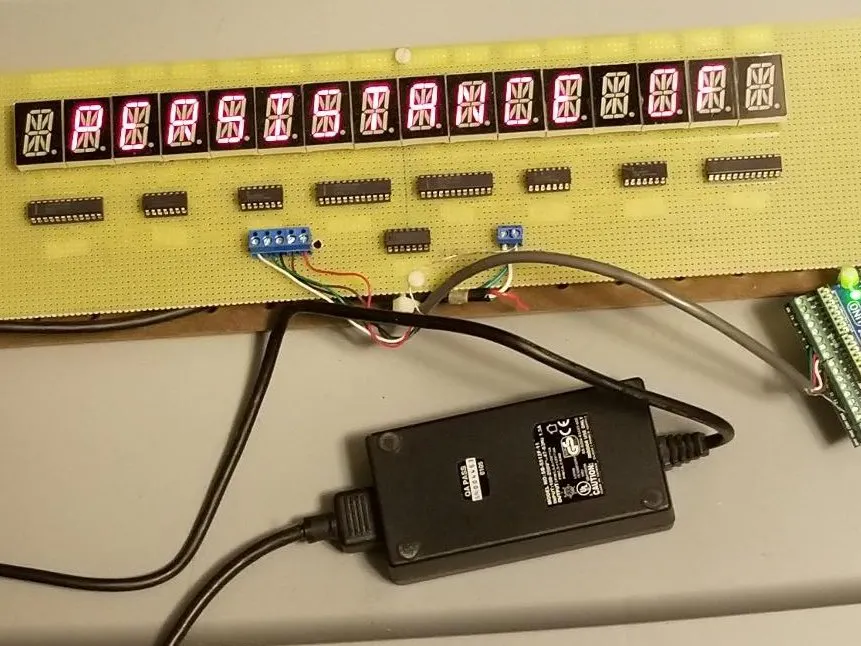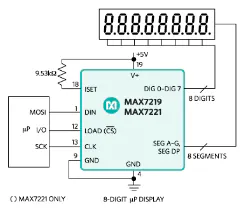# MAX7219 Conquers 16-Segment Display

The primitive AND gate provides a simple solution to driving 16-segments using 'persistence of vision'. Not fancy, just functional.## Things used in this project

### Hardware componentsMaxim Integrated MAX7219/MAX7221 LED Display Drivers
×4
 Texas Instruments 74LS08 Quad AND Gate
×1
 Texas Instruments 74LS09 Quad AND Gate
×4
 LED Display, 16-Segment, Common Cathode
×16

### Hand tools and fabrication machinesWire Wrap Tool, Gun

## Schematics

### 16-Segment Display Circuitry

Steering Logic on Sheet1, Drivers on Sheet2

### 16-Segment Common Cathode Display

This is what I used, however, any generic common cathode device should work as well.

### 74LS08 / 74LS09 Quad AND Gate

Any generic brand should work just fine, the pin-outs for the '08 and '09 are identical. The '08 is a standard TTL output, the '09 is an open-collector. These are not interchangeable!

### MAX7219 Display Driver

This is the workhorse of the project

### 16-Segment Wire Table

Provides the complete interconnection list for all components. This project uses 30AWG wire-wrap wire on wire-wrap IC sockets, all on a 17" x 4" perf board; somewhat primitive in today's world, but works for the Caveman.

## Code

### 16-Segment Display

Arduino
The Arduino UNO is the engine driving this project, powered from the USB connection to a desktop computer. The actual display circuitry is powered by a 5V, 5A desktop power supply. Interconnection between the Uno and display board requires 5 wires (clock, data, load, and two steering logic signals, LdOuter and LdInner). A ground is also required between the display board and Uno.
```#include <avr/pgmspace.h>
#include <stdlib.h>
const int NUMBER_OF_ELEMENTS = 96;
const int MAX_SIZE = 10;
const char OSeg [NUMBER_OF_ELEMENTS] [MAX_SIZE] PROGMEM = { //Outer Segment Codes
{"00000000"},  //
{"00000000"},  //
{"00000000"},  //
{"00000000"},  //
{"00000000"},  //
{"00000000"},  //
{"00000000"},  //
{"00000000"},  //
{"00000000"},  //
{"00000000"},  //
{"00000000"},  //*
{"00000000"},  //+
{"00000000"},  //
{"00000000"},  //-
{"00000000"},  //
{"00000000"},  ///
{"00111100"},  //0
{"00011000"},  //1
{"00110100"},  //2
{"00111100"},  //3
{"00011000"},  //4
{"00101100"},  //5
{"00101100"},  //6
{"00111000"},  //7
{"00111100"},  //8
{"00111100"},  //9
{"00000000"},  //
{"00000000"},  //
{"00000000"},  //
{"00000000"},  //
{"00000000"},  //
{"00000000"},  //
{"11110111"},  //@
{"11111001"},  //A
{"01111110"},  //B
{"11100111"},  //C
{"01111110"},  //D
{"11100111"},  //E
{"11100001"},  //F
{"11101111"},  //G
{"10011001"},  //H
{"01100110"},  //I
{"00011111"},  //J
{"10000001"},  //K
{"10000111"},  //L
{"10011001"},  //M
{"10011001"},  //N
{"11111111"},  //O
{"11110001"},  //P
{"11111111"},  //Q
{"11110001"},  //R
{"11101110"},  //S
{"01100000"},  //T
{"10011111"},  //U
{"10000001"},  //V
{"10011001"},  //W
{"00000000"},  //X
{"10011110"},  //Y
{"01100110"},  //Z
{"00100100"},  //[
{"00000000"},  //\
{"01000010"},  //]
{"00000000"},  //^
{"00000110"},  //_
{"00000000"},  //`
{"00000111"},  //a
{"10000011"},  //b
{"00000011"},  //c
{"00000011"},  //d
{"00000011"},  //e
{"00100000"},  //f
{"11000010"},  //g
{"10000001"},  //h
{"00000000"},  //i
{"00000011"},  //j
{"00000000"},  //k
{"00000000"},  //l
{"00001001"},  //m
{"00000001"},  //n
{"00000011"},  //o
{"11000001"},  //p
{"11000000"},  //q
{"00000001"},  //r
{"11000010"},  //s
{"10000011"},  //t
{"00000011"},  //u
{"00000001"},  //v
{"00001001"},  //w
{"00000000"},  //x
{"10000010"},  //y
{"00000010"},  //z
{"00100100"},  //{
{"00000000"},  //|
{"01000010"},  //}
{"00000000"},  //~
{"00000000"},  //

};

const char ISeg [NUMBER_OF_ELEMENTS] [MAX_SIZE] PROGMEM = {  // Inner Segment Codes
{"00000000"},  //
{"00000000"},  //
{"00000000"},  //
{"00000000"},  //
{"00000000"},  //
{"00000000"},  //
{"00000000"},  //
{"00000000"},  //
{"00000000"},  //
{"00000000"},  //
{"11111111"},  //*
{"10100101"},  //+
{"00000000"},  //
{"10000001"},  //-
{"00000000"},  //
{"00010010"},  ///
{"00100100"},  //0
{"00010000"},  //1
{"10000100"},  //2
{"10000000"},  //3
{"10100000"},  //4
{"10100000"},  //5
{"10100100"},  //6
{"00000000"},  //7
{"10100100"},  //8
{"10100000"},  //9
{"00000000"},  //
{"00000000"},  //
{"00000000"},  //
{"00000000"},  //
{"00000000"},  //
{"00000000"},  //
{"10100000"},  //@
{"10000001"},  //A
{"10100100"},  //B
{"00000000"},  //C
{"00100100"},  //D
{"00000001"},  //E
{"00000001"},  //F
{"10000000"},  //G
{"10000001"},  //H
{"00100100"},  //I
{"00000000"},  //J
{"00011001"},  //K
{"00000000"},  //L
{"01010000"},  //M
{"01001000"},  //N
{"00000000"},  //O
{"10000001"},  //P
{"00001000"},  //Q
{"10001001"},  //R
{"10000001"},  //S
{"00100100"},  //T
{"00000000"},  //U
{"00010010"},  //V
{"00001010"},  //W
{"01011010"},  //X
{"10000001"},  //Y
{"00010010"},  //Z
{"00100100"},  //[
{"01001000"},  //\
{"00100100"},  //]
{"00001010"},  //^
{"00000000"},  //_
{"01000000"},  //`
{"00000101"},  //a
{"00000101"},  //b
{"00000001"},  //c
{"00100101"},  //d
{"00000011"},  //e
{"10100101"},  //f
{"00100101"},  //g
{"00000101"},  //h
{"00000100"},  //i
{"00100100"},  //j
{"00111100"},  //k
{"00100100"},  //l
{"10000101"},  //m
{"00000101"},  //n
{"00000101"},  //o
{"00100001"},  //p
{"00100101"},  //q
{"00000001"},  //r
{"00000101"},  //s
{"00000001"},  //t
{"00000100"},  //u
{"00000010"},  //v
{"00001010"},  //w
{"01011010"},  //x
{"00100101"},  //y
{"00000011"},  //z
{"00100101"},  //{
{"00100100"},  //|
{"10100100"},  //}
{"10010011"},  //~
{"00000000"},  //

};

#define Interval_Message 1200
#define MAX7219_DATA 5
#define MAX7219_CLOCK 7
#define LdInner 9
#define LdOuter 8

unsigned long time1 = 0; // timer function
int x = 0; // message counter

// Various 16 character strings to display
const char string_0[] PROGMEM = " PERSISTANCE OF ";
const char string_1[] PROGMEM = "   VISION DEMO  ";
const char string_2[] PROGMEM = "USES Arduino Uno";
const char string_3[] PROGMEM = " Some AND gates ";
const char string_4[] PROGMEM = "MAX7219 and 16- ";
const char string_5[] PROGMEM = "Segment displays";
const char string_6[] PROGMEM = "                ";

// Load the above strings into progmem
const char *const string_table[] PROGMEM = {string_0,string_1,string_2,string_3,string_4,string_5,string_6};
char buffer;  // 16 characters + 1 null

void initialize()
{
pinMode(MAX7219_CLOCK,OUTPUT);
pinMode(MAX7219_DATA,OUTPUT);
pinMode(LdInner,OUTPUT);
pinMode(LdOuter,OUTPUT);
digitalWrite(LdInner, LOW);
digitalWrite(LdOuter,LOW);
}

// -------------------------------------------------------------------------------------------------------------------------------------------
void OuterSegments(byte address, word data) // Note: the 'data' is 16 bits which are split into highByte (for U6) and lowByte (for U2)
{
digitalWrite(LdOuter, HIGH); // AND Gate U1 sections A (clock signal) and D (load signal)

shiftOut(MAX7219_DATA, MAX7219_CLOCK, MSBFIRST, address); // U2 first gets the high byte (Upper 8 Displays Outer Segments) from the UNO
shiftOut(MAX7219_DATA, MAX7219_CLOCK, MSBFIRST, highByte(data));

shiftOut(MAX7219_DATA, MAX7219_CLOCK, MSBFIRST, address); // U2 now shifts the high byte into U6 while U2 gets the low byte (Lower 8 Displays Outer Segments)
shiftOut(MAX7219_DATA, MAX7219_CLOCK, MSBFIRST, lowByte(data));

digitalWrite(LdOuter, LOW);
}

void InnerSegments(byte address, word data) // Note: the 'data' is 16 bits which are split into highByte (for U9) and lowByte (for (U5)
{
digitalWrite(LdInner, HIGH); // AND Gate U1 sections B (clock signal) and C (load signal)

shiftOut(MAX7219_DATA, MAX7219_CLOCK, MSBFIRST, address); // U5 first gets the high byte (Upper 8 Displays Inner Segments) from the UNO
shiftOut(MAX7219_DATA, MAX7219_CLOCK, MSBFIRST, highByte(data));

shiftOut(MAX7219_DATA, MAX7219_CLOCK, MSBFIRST, address); // U5 now shifts the high byte into U9 while U5 gets the low byte (Lower 8 Displays Inner Segments)
shiftOut(MAX7219_DATA, MAX7219_CLOCK, MSBFIRST, lowByte(data));

digitalWrite(LdInner, LOW);
}

void CmdO(byte address, byte data) // these are U2 and U6 Control Register Commands (data is 8 bits)
{
digitalWrite(LdOuter, HIGH); // AND Gate U1 sections A (clock signal) and D (load signal)

shiftOut(MAX7219_DATA, MAX7219_CLOCK, MSBFIRST, address); // U2 first gets the address and data from the UNO
shiftOut(MAX7219_DATA, MAX7219_CLOCK, MSBFIRST, data);

shiftOut(MAX7219_DATA, MAX7219_CLOCK, MSBFIRST, address); // U2 shifts into U6 (Note: U2 and U6 get the identical address and data)
shiftOut(MAX7219_DATA, MAX7219_CLOCK, MSBFIRST, data);

digitalWrite(LdOuter, LOW);
}
void CmdI(byte address, byte data) // these are U5 and U9 Control Register Commands (data is 8 bits)
{
digitalWrite(LdInner, HIGH); // AND Gate U1 sections B (clock signal) and C (load signal)

shiftOut(MAX7219_DATA, MAX7219_CLOCK, MSBFIRST, address); // U5 first gets the address and data from the UNO
shiftOut(MAX7219_DATA, MAX7219_CLOCK, MSBFIRST, data);

shiftOut(MAX7219_DATA, MAX7219_CLOCK, MSBFIRST, address); // U5 shifts into U9 (Note: U5 and U9 get the identical address and data)
shiftOut(MAX7219_DATA, MAX7219_CLOCK, MSBFIRST, data);

digitalWrite(LdInner, LOW);
}

// --------------------------------------------------------------------------------------------------------------------------

void setup() {
// MAX7219 Control Register Set-Up

initialize();

// Outer Segment Set-Up (U2 and U6 - See MAX7219 Data Sheet page 7)
CmdO(0x0C, 0x00); // shutdown register
CmdO(0x0F, 0x00); // display test register - test mode off
CmdO(0x0B, 0x07); // scan limit register - display digits 0-7
CmdO(0x0A, 0x08); // intensity register - set display brightness
CmdO(0x09, 0x00); // decode mode register - set individual segments
CmdO(0x00, 0000); // No-Op

// Inner Segment Set-Up (U5 and U9 - See Max7219 Data Sheet page 7)
CmdI(0x0c, 0x00); // shutdown register
CmdI(0x0f, 0x00); // display test register - test mode off
CmdI(0x0b, 0x07); // scan limit register - display digits 0-7
CmdI(0x0a, 0x08); // intensity register - set display brightness
CmdI(0x09, 0x00); // decode mode register - set individual segments
CmdI(0x00, 0000); // No-Op
}

// ---------------------------------------------------------------------------------------------------------------------------------------------------

void get_message(int msg)
{
strcpy_P(buffer, (char *)pgm_read_word(&(string_table[msg])));  // get message string from progmem
String tempString = buffer;
char outSeg [MAX_SIZE];
char inSeg [MAX_SIZE];

for (int z = 0; z < 8; z++)
{
int get_asciiHOO = tempString.charAt(z);
memcpy_P (&outSeg, &OSeg [get_asciiHOO-32], sizeof outSeg); // Get the high order (Displays 9 thru 16) Outer Segment Codes from progmem
char *Lo1;
int OuterHigh = strtol(outSeg,&Lo1,2);

int get_asciiLOO = tempString.charAt(z+8);
memcpy_P (&outSeg, &OSeg [get_asciiLOO-32], sizeof outSeg); // Get the low order (Displays 1 thru 8) Outer Segment Codes from progmem
char *Lo2;
int OuterLow = strtol(outSeg,&Lo2,2);

word OUTERdata = 0; // create the 16 bit word where 'high' is display 16, 'low' is display 8; each iteration thru this loop decrements until 'high' is display 9 and 'low' is display 1
OUTERdata = word(OuterHigh,OuterLow);
OuterSegments(8-z,OUTERdata);

}
for (int z = 0; z < 8; z++)
{
int get_asciiHOI = tempString.charAt(z);
memcpy_P (&inSeg, &ISeg[get_asciiHOI-32], sizeof inSeg); // Get the high order (Displays 9 thru 16) Inner Segment Codes from progmem
char *Li1;
int InnerHigh = strtol(inSeg, &Li1,2);

int get_asciiLOI = tempString.charAt(z+8);
memcpy_P(&inSeg, &ISeg[get_asciiLOI-32],sizeof inSeg); // Get the low order (Displays 1 thru 8) Inner Segment Codes from progmem
char *Li2;
int InnerLow = strtol(inSeg, &Li2,2);

word INNERdata = 0;  // create the 16 bit word where 'high' is display 16, 'low' is display 8; each iteration thru this loop decrements until 'high' is display 9 and 'low' is display 1
INNERdata = word(InnerHigh,InnerLow);
InnerSegments(8-z,INNERdata);
}
}

void loop() {
// alternate inner segment chips (U5 and U9) and outer segment chips (U2 and U6) shutdown modes
// the human eye cannot perceive the switch between inner and outer segments
CmdO(0x0c,0001); // U2 and U6 exit Shutdown Mode
delay(10);
CmdO(0x0c,0000); // U2 and U6 enter Shutdown Mode
CmdI(0x0c,0001); // U5 and U9 exit Shutdown Mode
delay(10);
CmdI(0x0c,0000); // U5 and U9 enter Shutdown Mode

if(millis() > time1 + Interval_Message){
time1 = millis();
get_message(x);
x++;
if (x==7) x = 0;
}
}
```

## Credits

### OriginalCaveman

0 projects • 0 followers